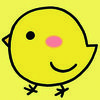/ 猿问

# 将列折叠/连接/聚合到每个组中的单个逗号分隔字符串

2019-05-25 16:20:07

data <- data.frame(A = c(rep(111, 3), rep(222, 3)), B = rep(1:2, 3), C = c(5:10))

data

#     A B  C

# 1 111 1  5

# 2 111 2  6

# 3 111 1  7

# 4 222 2  8

# 5 222 1  9

# 6 222 2 10

“A”和“B”是分组变量，“C”是我想要折叠成逗号分隔character字符串的变量。我试过了：

library(plyr)

ddply(data, .(A,B), summarise, test = list(C))

A B  test

1 111 1  5, 7

2 111 2     6

3 222 1     9

4 222 2 8, 10

ddply(data, .(A,B), summarise, test = as.character(list(C)))

#     A B     test

# 1 111 1  c(5, 7)

# 2 111 2        6

# 3 222 1        9

# 4 222 2 c(8, 10)

## 3 回答data.table

`# alternative using data.tablelibrary(data.table)as.data.table(data)[, toString(C), by = list(A, B)]`

aggregate这不使用包：

`# alternative using aggregate from the stats package in the core of Raggregate(C ~., data, toString)`

sqldf

`library(sqldf)sqldf("select A, B, group_concat(C) C from data group by A, B", method = "raw")`

dplyr`dplyr`替代：

```library(dplyr)data %>%
group_by(A, B) %>%
summarise(test = toString(C)) %>%
ungroup()```

plyr

`# plyrlibrary(plyr)ddply(data, .(A,B), summarize, C = toString(C))`> out <- ddply(data, .(A, B), summarise, test = list(as.character(C)))

> str(out)

'data.frame':   4 obs. of  3 variables:

\$ A   : num  111 111 222 222

\$ B   : int  1 2 1 2

\$ test:List of 4

..\$ : chr  "5" "7"

..\$ : chr "6"

..\$ : chr "9"

..\$ : chr  "8" "10"

> out

A B  test

1 111 1  5, 7

2 111 2     6

3 222 1     9

4 222 2 8, 10

> out2 <- ddply(data, .(A, B), summarise, test = paste(C, collapse = ", "))

> str(out2)

'data.frame':   4 obs. of  3 variables:

\$ A   : num  111 111 222 222

\$ B   : int  1 2 1 2

\$ test: chr  "5, 7" "6" "9" "8, 10"

> out

A B  test

1 111 1  5, 7

2 111 2     6

3 222 1     9

4 222 2 8, 10

> A1 <- aggregate(C ~ A + B, data, function(x) c(as.character(x)))

> str(A1)

'data.frame':   4 obs. of  3 variables:

\$ A: num  111 222 111 222

\$ B: int  1 1 2 2

\$ C:List of 4

..\$ 0: chr  "5" "7"

..\$ 1: chr "9"

..\$ 2: chr "6"

..\$ 3: chr  "8" "10"

> A2 <- aggregate(C ~ A + B, data, paste, collapse = ", ")

> str(A2)

'data.frame':   4 obs. of  3 variables:

\$ A: num  111 222 111 222

\$ B: int  1 1 2 2

\$ C: chr  "5, 7" "9" "6" "8, 10"library(tidyverse)

library(stringr)

data <- data.frame(A = c(rep(111, 3), rep(222, 3)), B = rep(1:2, 3), C = c(5:10))

data %>%

group_by(A, B) %>%

summarize(text = str_c(C, collapse = ", "))

# A tibble: 4 x 3

# Groups:   A 

A     B test

<dbl> <int> <chr>

1   111     1 5, 7

2   111     2 6

3   222     1 9

4   222     2 8, 10

• 3 回答
• 0 关注
• 151 浏览

0/150